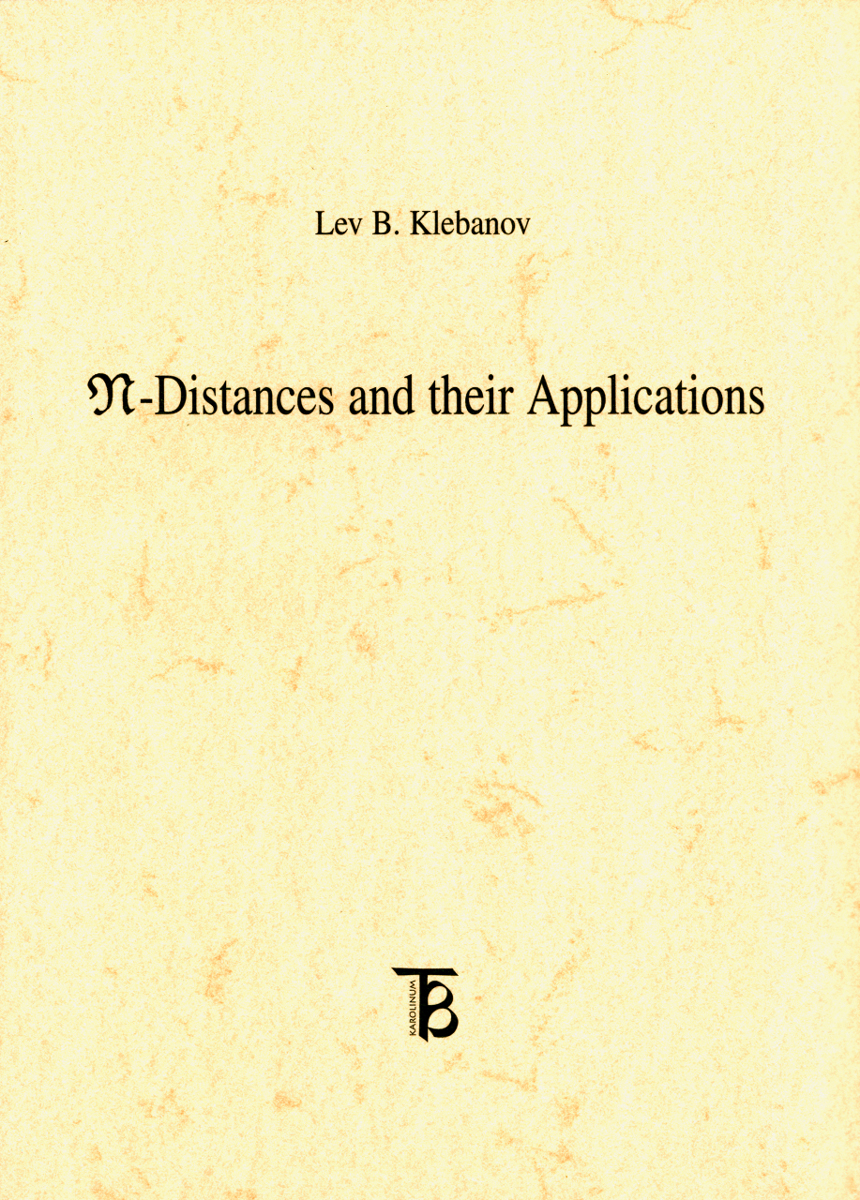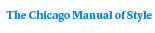# N-distances and Their Applications

Lev B. Klebanov### Distributed for Karolinum Press, Charles University

96 pages | 8 x 12
Paper \$15.00 ISBN: 9788024611525 Published April 2006 Not for sale in the Czech Republic and the Slovak Republic

The book focuses on probability metrics suitable for the characterization of random variables in Hilbert or Banach space. It provides details of various stochastic processes, such as testing non-deterministic statistical hypotheses, characterization of probability distribution or constructing multidimensional test for two selections. The book is published in the English language.

Table of Contents• Contents
Contents

Preface

Chapter 1. Positive and Negative Definite Kernels and Their Properties

1. Definitions of positive and negative definite kernels

2. Examples of positive definite kernels

3. Positive definite functions

4. Negative definite kernels

5. Coarse embeddings of metric spaces into Hilbert space

6. Strictly and strongly positive and negative definite kernels

Chapter 2. N-Metrics in the Set of Probability Measures

1. A class of positive definite kernels in the set of probabilities and N-distances

Chapter 3. m-negative Definite Kernels and Metrics

Chapter 4. N-metrics and the Problem of Recovering Measures From Potential

1. Recovering Measures From Potential

2. Stability in the Problem of Recovering a Measure from Potential

Chapter 5. N-metrics in the Study of Certain Problems of Characterization of Distributions

1. Some characterization of Gaussian and related distributions

2. Characterization of distributions symmetric to a group of transformations

Chapter 6. Commutative Semigroups with Positive Definite Kernel

1. General considerations

2. Distances in X

3. Special representations

4. Properties of x(t)

5. Infinitely divisible elements

6. Accompanying infinitely divisible elements

7. Examples

Chapter 7. Statistical Estimates obtained by the Minimal Distances Method

1. Estimating a location parameter, I

2. Estimating a location parameter, II

3. Estimating a general parameter

4. Estimating a location parameter, III

5. Semiparametric estimation

Chapter 8. Some Statistical Tests Based on N-Distances

1. Multivariate two-sample test

2. Test for two distributions to belong to the same additive type

3. Some Tests for Observations to be Gaussian

4. A Test for Closeness of Probability Distributions

Chapter 9. A Permutation Test Motivated by Microarray Data Analysis 1. Introduction

2. Theoretical framework: the test statistic

3. Computational framework: accuracy of permutation quantiles and p-values

4. Power of the test: a simulation study

5. Examples of data analysis

6. Conclusions of the Chapter

Bibliography

Author Index

Index